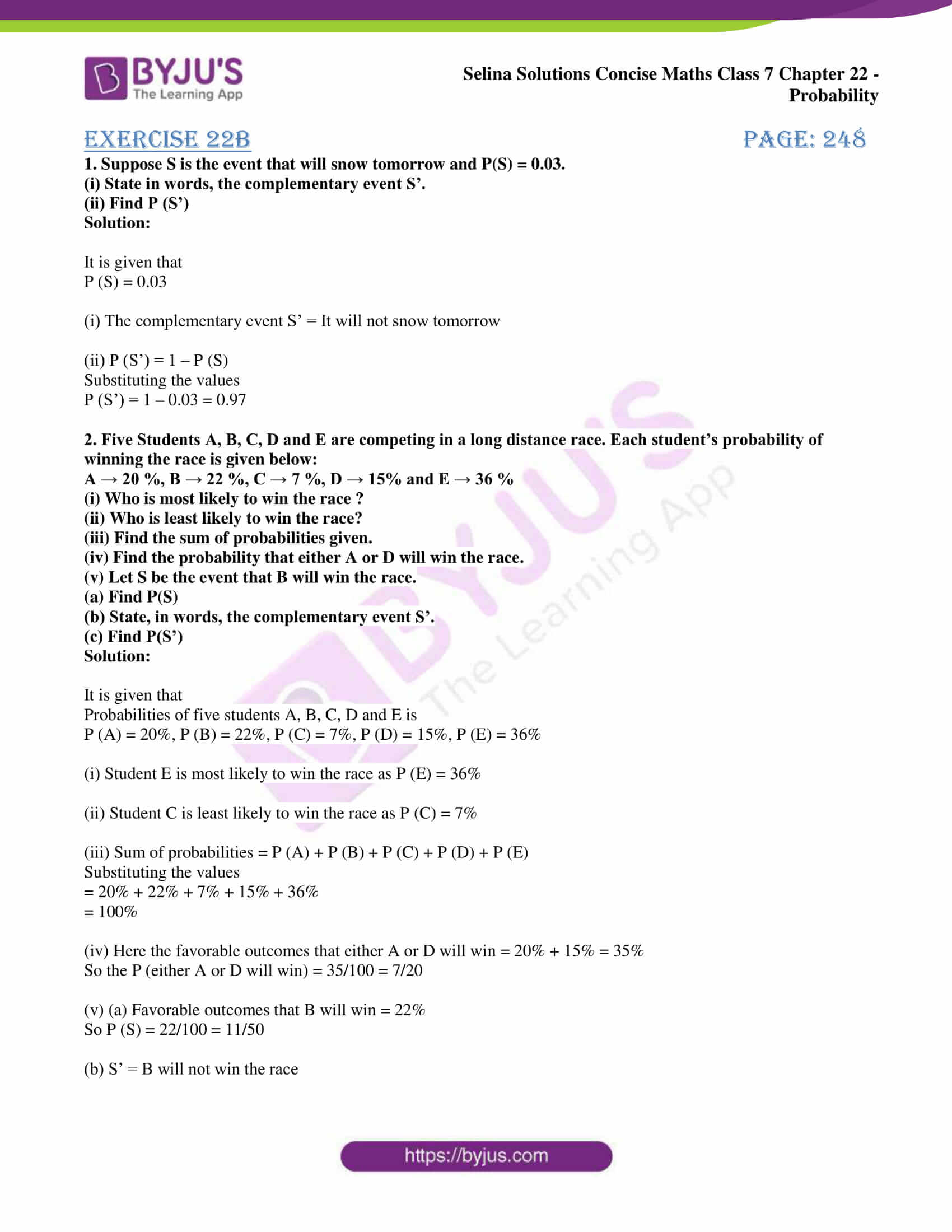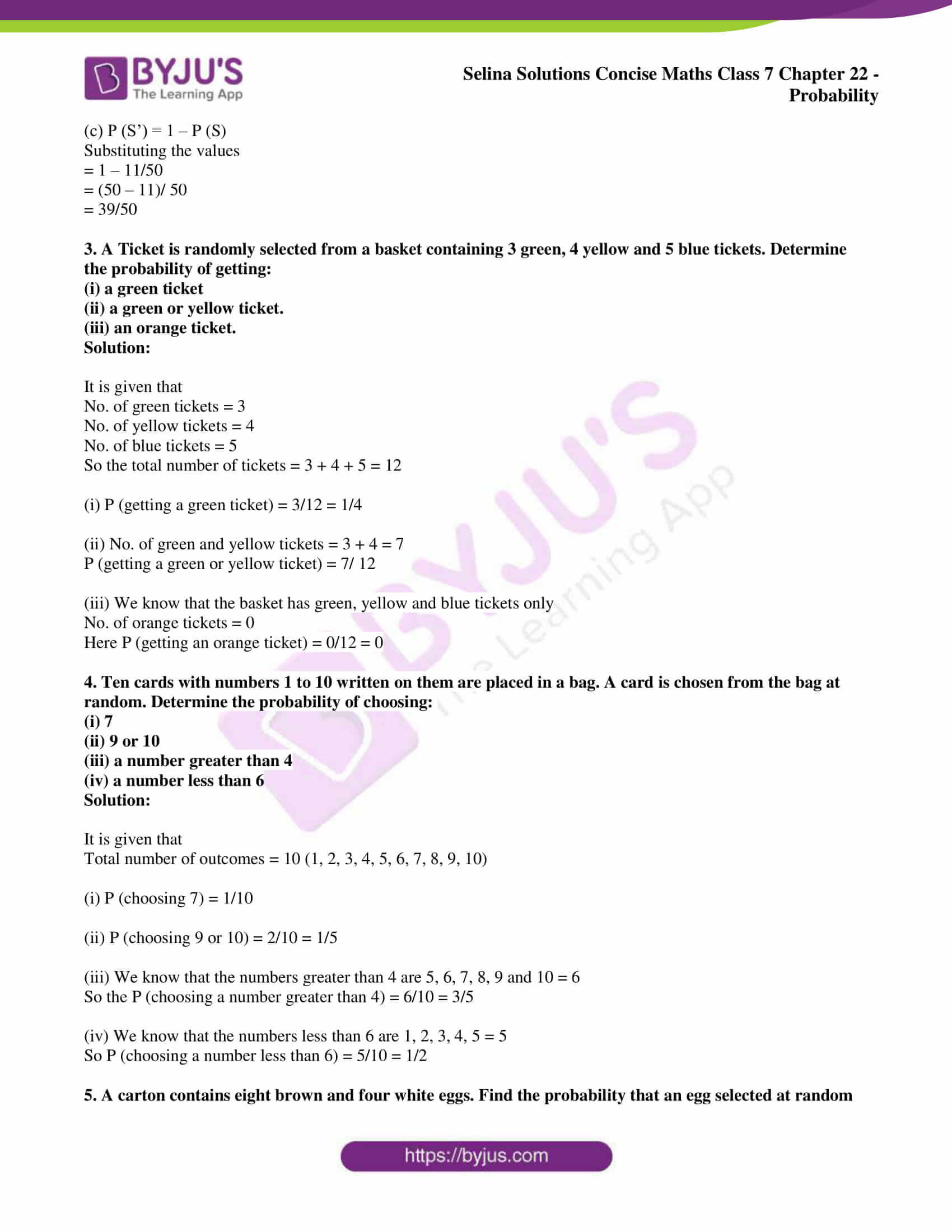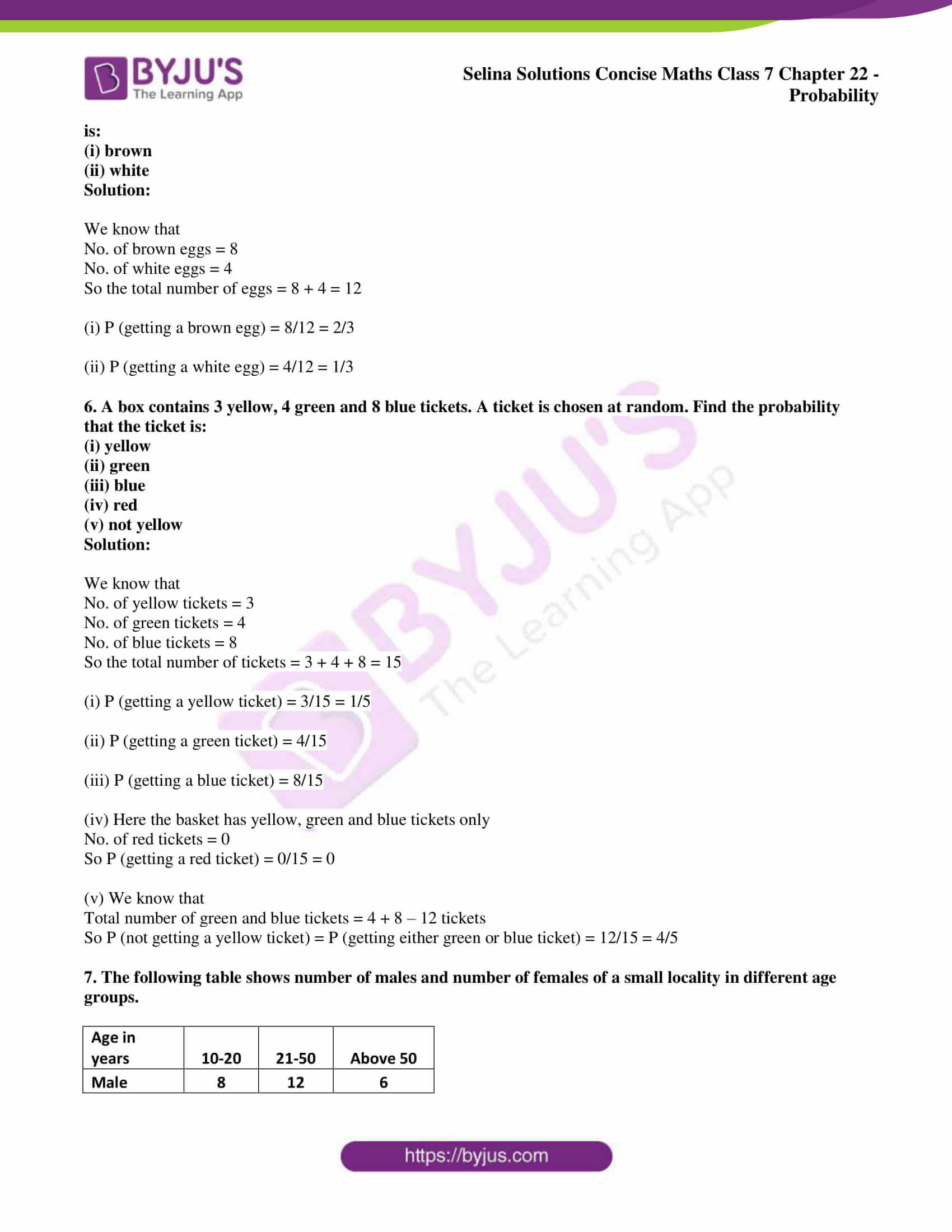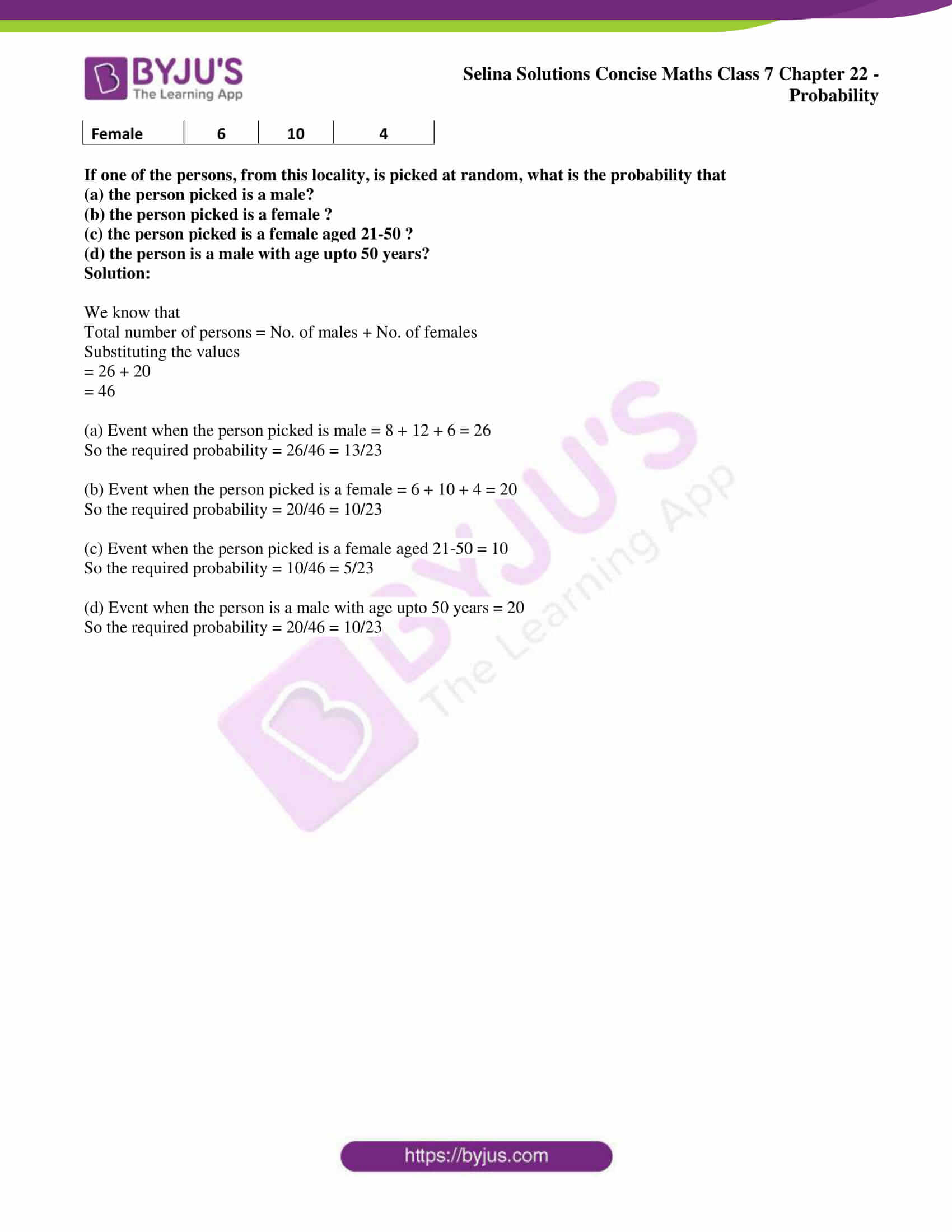# Selina Solutions Concise Maths Class 7 Chapter 22: Probability Exercise 22B

Selina Solutions Concise Maths Class 7 Chapter 22 Probability Exercise 22B are made available in PDF format for the convenience of students. Scoring marks in a subject like Mathematics is very easy as it mainly requires regular practise. It mainly improves analytical and logical thinking abilities among students to solve tricky problems effortlessly. PDF has important shortcut methods of solving problems in a shorter duration. Here, the students can download Selina Solutions Concise Maths Class 7 Chapter 22 Probability Exercise 22B PDF, from the links which are available below.

## Selina Solutions Concise Maths Class 7 Chapter 22: Probability Exercise 22B Download PDF### Access Selina Solutions Concise Maths Class 7 Chapter 22: Probability Exercise 22B

#### Exercise 22B page: 248

1. Suppose S is the event that will snow tomorrow and P(S) = 0.03.

(i) State in words, the complementary event S’.

(ii) Find P (S’)

Solution:

It is given that

P (S) = 0.03

(i) The complementary event S’ = It will not snow tomorrow

(ii) P (S’) = 1 – P (S)

Substituting the values

P (S’) = 1 – 0.03 = 0.97

2. Five Students A, B, C, D and E are competing in a long distance race. Each student’s probability of winning the race is given below:

A → 20 %, B → 22 %, C → 7 %, D → 15% and E → 36 %

(i) Who is most likely to win the race ?

(ii) Who is least likely to win the race?

(iii) Find the sum of probabilities given.

(iv) Find the probability that either A or D will win the race.

(v) Let S be the event that B will win the race.

(a) Find P(S)

(b) State, in words, the complementary event S’.

(c) Find P(S’)

Solution:

It is given that

Probabilities of five students A, B, C, D and E is

P (A) = 20%, P (B) = 22%, P (C) = 7%, P (D) = 15%, P (E) = 36%

(i) Student E is most likely to win the race as P (E) = 36%

(ii) Student C is least likely to win the race as P (C) = 7%

(iii) Sum of probabilities = P (A) + P (B) + P (C) + P (D) + P (E)

Substituting the values

= 20% + 22% + 7% + 15% + 36%

= 100%

(iv) Here the favorable outcomes that either A or D will win = 20% + 15% = 35%

So the P (either A or D will win) = 35/100 = 7/20

(v) (a) Favorable outcomes that B will win = 22%

So P (S) = 22/100 = 11/50

(b) S’ = B will not win the race

(c) P (S’) = 1 – P (S)

Substituting the values

= 1 – 11/50

= (50 – 11)/ 50

= 39/50

3. A Ticket is randomly selected from a basket containing 3 green, 4 yellow and 5 blue tickets. Determine the probability of getting:

(i) a green ticket

(ii) a green or yellow ticket.

(iii) an orange ticket.

Solution:

It is given that

No. of green tickets = 3

No. of yellow tickets = 4

No. of blue tickets = 5

So the total number of tickets = 3 + 4 + 5 = 12

(i) P (getting a green ticket) = 3/12 = 1/4

(ii) No. of green and yellow tickets = 3 + 4 = 7

P (getting a green or yellow ticket) = 7/ 12

(iii) We know that the basket has green, yellow and blue tickets only

No. of orange tickets = 0

Here P (getting an orange ticket) = 0/12 = 0

4. Ten cards with numbers 1 to 10 written on them are placed in a bag. A card is chosen from the bag at random. Determine the probability of choosing:

(i) 7

(ii) 9 or 10

(iii) a number greater than 4

(iv) a number less than 6

Solution:

It is given that

Total number of outcomes = 10 (1, 2, 3, 4, 5, 6, 7, 8, 9, 10)

(i) P (choosing 7) = 1/10

(ii) P (choosing 9 or 10) = 2/10 = 1/5

(iii) We know that the numbers greater than 4 are 5, 6, 7, 8, 9 and 10 = 6

So the P (choosing a number greater than 4) = 6/10 = 3/5

(iv) We know that the numbers less than 6 are 1, 2, 3, 4, 5 = 5

So P (choosing a number less than 6) = 5/10 = 1/2

5. A carton contains eight brown and four white eggs. Find the probability that an egg selected at random is:

(i) brown

(ii) white

Solution:

We know that

No. of brown eggs = 8

No. of white eggs = 4

So the total number of eggs = 8 + 4 = 12

(i) P (getting a brown egg) = 8/12 = 2/3

(ii) P (getting a white egg) = 4/12 = 1/3

6. A box contains 3 yellow, 4 green and 8 blue tickets. A ticket is chosen at random. Find the probability that the ticket is:

(i) yellow

(ii) green

(iii) blue

(iv) red

(v) not yellow

Solution:

We know that

No. of yellow tickets = 3

No. of green tickets = 4

No. of blue tickets = 8

So the total number of tickets = 3 + 4 + 8 = 15

(i) P (getting a yellow ticket) = 3/15 = 1/5

(ii) P (getting a green ticket) = 4/15

(iii) P (getting a blue ticket) = 8/15

(iv) Here the basket has yellow, green and blue tickets only

No. of red tickets = 0

So P (getting a red ticket) = 0/15 = 0

(v) We know that

Total number of green and blue tickets = 4 + 8 – 12 tickets

So P (not getting a yellow ticket) = P (getting either green or blue ticket) = 12/15 = 4/5

7. The following table shows number of males and number of females of a small locality in different age groups.

 Age in years 10-20 21-50 Above 50 Male 8 12 6 Female 6 10 4

If one of the persons, from this locality, is picked at random, what is the probability that
(a) the person picked is a male?
(b) the person picked is a female ?
(c) the person picked is a female aged 21-50 ?
(d) the person is a male with age upto 50 years?

Solution:

We know that

Total number of persons = No. of males + No. of females

Substituting the values

= 26 + 20

= 46

(a) Event when the person picked is male = 8 + 12 + 6 = 26

So the required probability = 26/46 = 13/23

(b) Event when the person picked is a female = 6 + 10 + 4 = 20

So the required probability = 20/46 = 10/23

(c) Event when the person picked is a female aged 21-50 = 10

So the required probability = 10/46 = 5/23

(d) Event when the person is a male with age upto 50 years = 20

So the required probability = 20/46 = 10/23